Tamilnadu State Board New Syllabus Samacheer Kalvi 11th Computer Science Guide Pdf Chapter 7 Composition and Decomposition Text Book Back Questions and Answers, Notes.

Tamilnadu Samacheer Kalvi 11th Computer Science Solutions Chapter 7 Composition and Decomposition

11th Computer Science Guide Composition and Decomposition Text Book Questions and AnswersPart I

Question 1.
Suppose u, v = 10, 5 before the assignment. What are the values of u and v after the . sequence of assignments?
1, u := v
2. v := u
a) u, v = 5, 5
b) u, v = 5, 10
c) u, v = 10, 5
d) u, v = 10, 10
a) u, v = 5, 5

Question 2.
Which of the following properties is true after the assignment (at line 3)?
1. — i + j = 0
2. i, j : = i + 1, j – 1
3. –?
a) i + j >0
b) i + j < 0
c) i + j = 0
d) i = j
b) i + j < 0Question 3.
If Cl is false and C2 is true, the compound statement,
1. if C1
2. S1
3. else
4. if C2
5. S2
6. else
7. S3
executes
a) S1
b) S2
c) S3
d) none
b) S2

Question 4.
If C is false just before the loop, the control flows through. –
1. S1
2. while C
3. S2
4. S3
a) S1 ; S3
b) S1 ; S2 ; S3
c) S1 ; S2 ; S2 ; S3
d) S1; S2 ; S2 ; S2 ; S3
a) S1 ; S3

Question 5.
If C is true, S1 is executed in both the flowcharts, but S2 is executed in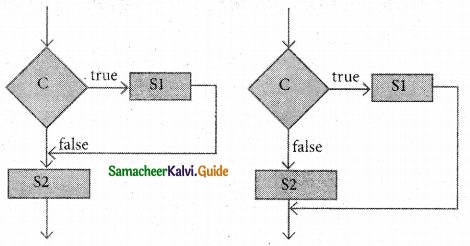a) (1) only
b) (2) only
c) both (1) and (2)
d) neither (1) nor (2)
a) (1) onlyQuestion 6.
How many times the loop is iterated? i := 0
while i ≠ 5
i : = i + 1
a) 4
b) 5
c) 6
d) 0
b) 5

Part II

Question 1.
Distinguish between a condition and a statement.

 Condition Statement It is a phrase that describes a test of the state. It is a phrase that commands the computer to do an action.

Question 2.
Draw a flowchart for a conditional statement.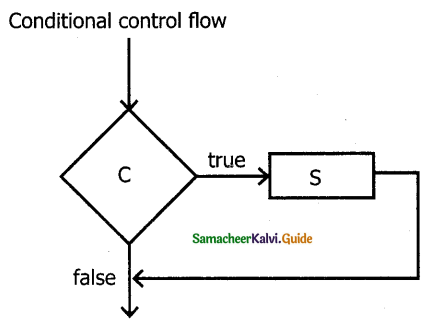Question 3.
Both conditional statements and iterative statements have a condition and a statement. How do they differ?
Conditional Statement:

• Statements are executed only once when the condition is true.
• If condition statement.

Iterative Statement:

• An iterative statement repeatedly evaluates a condition and executes a statement until it becomes false.
• While condition statement.

Question 4.
What is the difference between an algorithm and a program?

 ALGORITHM PROGRAM It is a step-by-step procedure to solve a problem. It is a set of instructions to solve a problem by the computer. No need to follow the grammar of a language Follow strictly the grammar of a programming language.

Question 5.
Why is a function an abstraction?
Once a function is defined, it can be used over and over and over again. Reusability of a single function several times is known as an abstraction.

Question 6.
How do we define a statement?
In a refinement, starting at a high level, each statement is repeatedly expanded into more detailed statements in the subsequent levels.Part III

Explain in brief

Question 1.
For the given two flowcharts write the pseudo-code.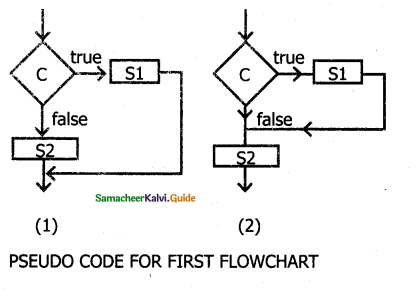if condition is True
Execute Statement S1
else
Execute Statement S2
endif
PSEUDO CODE FOR SECOND FLOWCHART
if the condition is True
Execute Statement S1
Execute Statement S2
else
Execute Statement S2
endif.Question 2.
If C is false in line 2, trace the control flow in this algorithm.
1. S1
2. — C is false
3. if C
4. S2
5. else
6. S3
7. S4
The control flow for the given algorithm is as follows:
S1
S3
S4
The condition is false so it executes S3. In this case S2 skipped.Question 3.
What is case analysis?
The case Analysis statement generalizes the problem into multiple cases. Case Analysis splits the problem into an exhaustive set of disjoint cases.

Question 4.
Draw a flowchart for -3 case analysis using alternative statements.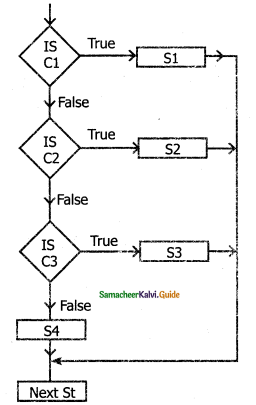Where C1, C2 and C3 are conditions
S1, S2, S3 and S4 are statements.Question 5.
Define a function to double a number in two different ways: (1) n + n,(2)2 x n.
1. Double (n)
– – inputs: n is a real number or an integer, n > 0
– – Outputs: y is a real number or an integer such that y = n + n

2. Double (n)
– – inputs: n is a real number or an integer, n > 0
– – Outputs: y is a real number or an integer such that y = 2 x n

Part IV

Explain in detail

Question 1.
Exchange the contents: Given two glasses marked A and B. Glass A is full of apple drink and glass B is full of grape drink. Write the specification for exchanging the contents of glasses A and B, and write a sequence of assignments to satisfy the specification.
The sequence of assignments to satisfy the specification:
exchange(A, B)
–inputs : A, B are real and > 0
–outputs: A, B are real and > 0
State representation
T = A
A: = B
B: = TQuestion 2.
Circulate the contents: Write the specification and construct an algorithm to circulate the contents of the variables A, B and C as shown below: The arrows indicate that B gets the value of A, C gets the value of B and A gets the value of C.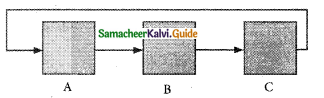Specification :
Circulated,B,C)
– – inputs: A,B,C all are real numbers
– – outputs: A,B,C, all are real numbers
T: = C
C: = B
B: = A
A: = T

Algorithm :
i) circulated,B,C)
ii) – – A,B,C
iii) T = C
iv) C = B
v) B = A
vi) A: = T

Question 3.
Decanting problem. You are given three bottles of capacities 5, 8, and 3 litres. The 8L bottle is filled with oil, while the other two are empty. Divide the oil in an 8L bottle into two equal quantities. Represent the state of the process by appropriate variables. What are the initial and final states of the process? Model the decanting of oil from one bottle to another by assignment. Write a sequence of assignments to achieve the final state.
To divide the oil equally in the oil bottle, the following 7 steps are needed:

• Pour 5L of oil into the second bottle. (3,5,0)
• Pour 3L from the second bottle to the third. (3,2,3)
• Pour 3L from the third to the first. (6,2,0)
• Pour all 2L from the second to the third bottle (6,0,2)
• Pour 5L from the first to the second bottle. (1.5.2)
• Pour 1 L from the second to the third bottle. (1.4.3)
• Finally, pour now all 3L from the third bottle to the first (4,4,0)Assignment statement as follows:

• E,F,T = 8,0,0
• E,F,T = 3,5,0
• E,F,T = 3,2,3
• E,F,T = 6,2,0
• E,F,T = 6,0,2
• E,F,T = 1,5,2
• E,F,T = 1,4,3
• E,F,T = 4,4,0

Question 4.
Trace the step-by-step execution of the algorithm for factorial(4).
factorial(n)
— inputs i rs is an integer, n > 0
— outputs : f ‘=s n!
f, i := 1,1
while i ≤ n
f,i = 11= f x i, i+1
Tracing steps:
factorial(5)
i) f = 1, i = 1
ii) f = 1 x 1, i = 2
iii) f = 1 x 2, i = 3
iv) f = 2 x 3, i = 4
v) f = 6 x 4, i = 5
vi) f = 24 x 5, i = 6
vii) condition 6 < 5 becomes false then display output f value as 24.

Part I

Question 1.
Which one of the following is odd?
(a) Python
(b) C++
(c) C
(d) Ctrl + S
(d) Ctrl + SQuestion 2.
_________ is a notation for representing algorithms.
a) programming language
b) pseudo code
c) flow chart
d) All the above
d) All the above

Question 3.
There are important control flow statements.
(a) four
(b) three
(c) two
(d) five
(b) three

Question 4.
_________ is a notation similar to programming languages.
a) programming language
b) pseudo code
c) flow chart
d) none of these
b) pseudo code

Question 5.
A ………………. is contained in a rectangular box with a single outgoing arrow, which points to the box to be executed next.
(a) statement
(b) composition
(c) notation
(d) condition
(a) statementQuestion 6.
_________ is a diagrammatic notation for representing algorithms.
a) programming language
b) pseudo code
c) flow chart
d) none of these
c) flow chart

Question 7.
The algorithm can be specified as ……………….
(a) monochromatize (a, b, c)
(b) a = b = 0
(c) C = A + B + C
(d) none
(a) monochromatize (a, b, c)

Question 8.
An algorithm expressed in a_________ is called a program.
a) programming language
b) pseudo code
c) flow chart
d) none of these
a) programming language

Question 9.
Which one of the following is the elementary problem-solving technique?
(a) Specification
(b) Abstraction
(c) Composition
(d) decomposition
(d) decomposition

Question 10.
_________ is formal.
a) programming language
b) pseudo code
c) flow chart
d) none of these
a) programming language

Question 11.
How many different notations are there for representing algorithms?
(a) 2
(b) 3
(c) 4
(d) 5
(b) 3Question 12.
_________ do not allow the informal style of natural languages such as English or Tamil.
a) programs
b) pseudo code
c) flow charts
d) none of these
a) programs

Question 13.
Which one of the following algorithmic notations is used for communication among people?
(a) Flow chart
(b) Pseudo code
(c) PL
(d) Interpreter
(b) Pseudo code

Question 14.
_________ notation is not formal nor exact.
a) programs
b) pseudo code
c) flow charts
d) none of these
b) pseudo code

Question 15.
The algorithmic notation similar to a Programming language is ……………….
(a) Flow chart
(b) Pseudo code
(c) C ++
(d) C
(b) Pseudo codeQuestion 16.
In _________, there is no need to follow the rules of the grammar of a programming language.
a) programs
b) pseudo code
c) flow charts
d) none of these
b) pseudo code

Question 17.
Which one is used for converting programs into computer-executable instructions?
(a) Converter
(b) Apps
(c) Translator
(d) exe files
(c) Translator

Question 18.
_________ show the control flow of algorithms using diagrams in a visual manner.
a) programs
b) pseudo code
c) flow charts
d) none of these
c) flow charts

Question 19.
The notation which is not formal nor exact is ……………….
(a) Flow chart
(b) Pseudocode
(c) Compiler
(d) Translator
(b) Pseudocode

Question 20.
In flowcharts, _________ boxes represent conditions.
a) arrows
b) diamond shaped
c) rectangular shaped
d) none of these
b) diamond shapedQuestion 21.
Find the pair which is wrongly matched.
(a) Rectangular boxes – Statements
(b) Diamond boxes – Output
(c) Arrow – Control flow
(d) Parallelogram – Input
(b) Diamond boxes – Output

Question 22.
A condition is contained in a diamond-shaped box with _________ outgoing arrows.
a) two
b) three
c) one
d) many
a) two

Question 23.
The inputs and outputs are drawn using ……………….. boxes.
(a) rectangular
(b) diamond
(c) Parallelogram
(d) Oval
(c) Parallelogram

Question 24.
In flow chart, _________ marked Start and the End are used to indicate the start and the end of an execution:
a) special box (oval)
b) diamond shaped
c) rectangular shaped
d) parallelogram
a) special box (oval)Question 25.
The flow of control is represented in the flowchart by ………………..
(a) arrow
(b) dot
(c) box
(d) plus
(a) arrow

Question 26.
A_________ is a phrase that commands the computer to do an action.
a) statement
b) sentence
c) walkthrough
d) none of these
a) statement

Question 27.
A condition is contained in a diamond-shaped box with ……………….. outgoing arrows.
(a) 2
(b) 3
(c) 4
(d) 5
(a) 2

Question 28.
Statements composed of other statements are known as_________ statements.
a) compound
b) bock
c) nested
c) none of these
a) compound

Question 29.
_________ statement are compound statements.
a) input
b) output
c) comment
d) control flow
d) control flowQuestion 30.
How many outgoing arrows are needed for rectangular boxes in the flow chart?
(a) 0
(b) 1
(c) 2
(d) 3
(b) 1

Question 31.
___________ is a control flow statements.
a) Sequential
b) Alternative
c) Iterative
d) All the above
d) All the above

Question 32.
Which one of the following is not a control flow statement?
(a) Sequential
(b) Assignment
(c) Iterative
(d) Alternative
(b) Assignment

Question 33.
Case analysis splits the problem into an exhaustive set of __________ cases.
a) disjoint
b) similar
c) recursive
d) none of these
a) disjointQuestion 34.
Which one of the following statements are executed one after the other as written in the algorithm?
(a) Sequential
(b) Iterative
(c) Conditional
(d) Decisive
(a) Sequential

Question 35.
An__________ executes the same action repeatedly, subject to a condition.
a) iterative process
b) condition
c) sequential
d) None of these
a) iterative process

Question 36.
Case analysis statement generalizes the statement into ……………….. cases.
(a) 2
(b) 3
(c) 5
(d) multiple
(d) multiple

Question 37.
Testing the loop condition and executing the , loop body once is called.
a) iteration
b) condition
c) sequential
d) none of these
a) iterationQuestion 38.
Which one of the following processes executes the same action repeatedly?
(a) Conditional
(b) Alternative
(c) Iterative
(d) None of these
(c) Iterative

Question 39.
__________ breaking and combining the solutions of the smaller problems to solve the original problem.
a) Composition
b) Decomposition
c) Eliminating
d) None of these
b) Decomposition

Question 40.
Testing the loop condition and executing the loop body once is called ………………..
(a) alternative
(b) conditional
(c) Iteration
(d) Decomposition
(c) Iteration

Question 41.
A(n)__________ is like a sub-algorithm.
a) function
b) array
c) structure
d) None of these
a) functionQuestion 42.
A __________ can be used as a black box in solving other problems.
a) function
b) array
c) structure
d) None of these
a) function

Question 43.
Identify the correct statement from the following.
a) There is no need for the users to know how the function is implemented in order to use it.
b) An algorithm used to implement a function may maintain its own variables.
c) users of the function need only to know what the function does, and not how it is done by the function.
d) All the above
d) All the above

Question 44.
Conditional statement is executed only if the condition is true. Otherwise, __________ .
a) terminates the algorithm
b) repeat the procedure
c) skip the loop
d) nothing is done
d) nothing is done

Part II

Question 1.
What is programming language?
A programming language is a notation for expressing algorithms to be executed by computers.

Question 2.
What is a Pseudocode?
Pseudocode is a mix of programming languages like constructs and plain English. Algorithms expressed in Pseudocode are not intended to be executed by computers but for human readers to understand.

Question 3.
What is flowchart?
A flowchart is a diagrammatic notation for representing algorithms. They give a visual intuition of the flow of control, when the algorithm is executed.Question 4.
What is a control flow statement? Classify it.
Control flow statements are compound statements. They are used to alter the control flow of the process depending on the state of the process. They are classified as:

1. Sequential
2. Alternative
3. Iterative

Question 5.
What are the constraints of a programming language?

• Programming language is formal.
• Programs must obey the grammar of the programming language exactly. Even punctuation symbols must be exact.
• They do not allow the informal style of natural languages such as English or Tamil.

Question 6.
When a condition statement will be executed?
It will be executed only when the condition statement is true.

Question 7.
What is the advantage of a flowchart?
They show the control flow of algorithms using diagrams in a visual manner.

Question 8.
What is a statement?
A statement is a phrase that commands the computer to do an action.Question 9.
What do you mean by compound statement?
Statements may be composed of other statements, leading to a hierarchical structure of algorithms. Statements composed of other statements are known as compound statements.

Question 10.
What are the control flow statements?
There are three important control flow statements:

• Sequential
• Alternative
• Iterative

Question 11.
What happen when a control flow statement is executed?
When a control flow statement is executed, the state of the process is tested, and depending on the result, a statement is selected for execution.

Question 12.
Write the specification for finding minimum of two numbers.
The specification of algorithm minimum is minimum (a, b)
– – inputs: a , b
– – outputs: result = a ↓ b.

Part III

Explain in brief

Question 1.
How many notations are there for representing algorithms? Explain.
There are mainly three different notations for representing algorithms.

• A programming language is a notation for expressing algorithms to be executed by computers.
• Pseudocode is a notation similar to programming languages. Algorithms expressed in pseudo-code are not intended to be executed by computers, but for communication among people.
• A flowchart is a diagrammatic notation for representing algorithms. They give a visual intuition of the flow of control, when the algorithm is executed.

Question 2.
What are the disadvantages of a flowchart?
Flowcharts also have the following disadvantages:

• Flowcharts are less compact than the representation of algorithms in a programming language or pseudo code.
• They obscure the basic hierarchical structure of the algorithms.
• Alternative statements and loops are disciplined control flow structures.Question 3.
Write a note on refinement.
After decomposing a problem into smaller subproblems, the next step is either to refine the subproblem or to abstract the subproblem.
1. Each subproblem can be expanded into more detailed steps. Each step can be further expanded to still finer steps, and so on. This is known as refinement.

2. We can also abstract the subproblem. We specify each subproblem by its input property and the input-output relation. While solving the main problem, we only need to know the specification of the subproblems. We do not need to know how the subproblems are solved.

Question 4.
Write an algorithm that compares numbers and produces the result as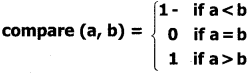We can split the state into an exhaustive set of 3 disjoint cases: a < b, a = b, and a> b. Then we can define compare() using a case analysis. The algorithm is as given below.

1. compare(a, b)
2. case a < b
3. result := -1
4. case a = b
5. result := 0
6. else – – a > b
7. result: = 1

Question 5.
Construct specification and write an iterative algorithm to compute the quotient and remainder after dividing an integer A by another integer B.
Specification: divide (A, B)
— inputs: A is an integer and B ≠ 0
— outputs : q and r such that A = q x B
+ r and 0 ≤ r < B

Algorithm:
divide (A, B)
— inputs: A is an integer and B * 0
— outputs : q and r such that
A = q X B + r and
— 0 < r < B
q := 0, A
while r ≥ B
q, r := q + 1, r – B

Question 6.
Explain Decomposition.
It involves breaking down a problem into smaller and more manageable problems, and combining the solutions of the smaller problems to solve the original problem. Often, problems have structure.
We can exploit the structure of the problem and break it into smaller problems. Then, the smaller problems can be further broken until they become sufficiently small to be solved by other simpler means.
Their solutions are then combined together to construct a solution to the original problem.Question 7.
Construct an iterative algorithm to compute the quotient and remainder after dividing an integer A by another integer B.
divide (A, B)
– – inputs: A is an integer and B ≠ 0
– – Outputs: q and r such that A = q x B + r and 0 < r < B
q : = 0, A
While r ≥ B
q, r : = q + 1, r – B

Question 8.
Draw a flow chart for Eat breakfast.
Flowchart for Eat breakfast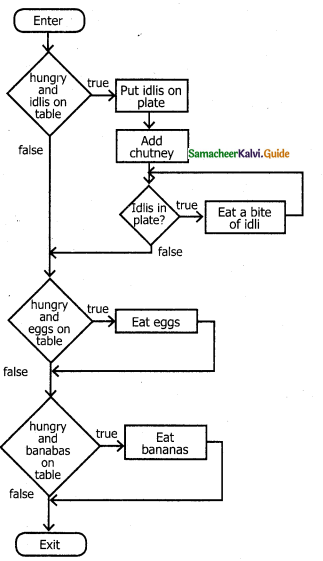Part IV

Explain in detail

Question 1.
What is flowchart? Explain the various boxes (symbols) used in flowchart.
A flowchart is a diagrammatic notation for representing algorithms. They show the control flow of algorithms using diagrams in a visual manner.

In flowcharts, rectangular boxes represent simple statements, diamond-shaped boxes
represent conditions, and arrows describe how the control flows during the execution of the algorithm. A flowchart is a collection of boxes containing statements and conditions which are connected by arrows showing the order in which the boxes are to be executed,

i) A statement is contained in a rectangular box with a single outgoing arrow, which points to the box to be executed next.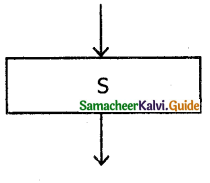ii) A condition is contained in a diamond-shaped box with two outgoing arrows, labeled true and false. The true arrow points to the box to be executed next if the condition is true, and the false arrow points to the box to be executed next if the condition is false.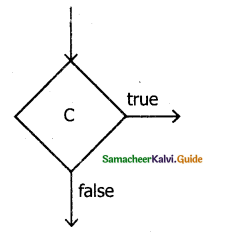iii) Parallelogram boxes represent inputs given and outputs produced.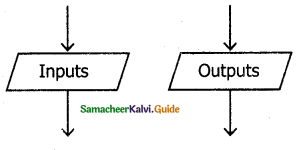iv) Special boxes marked Start and the End is used to indicate the start and the end of execution.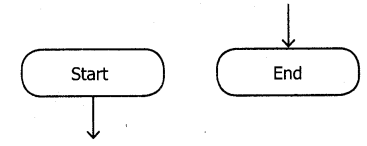Question 2.
Draw a flowchart to compute the quotient and remainder after dividing an integer A by another integer B.
Flowchart for integer division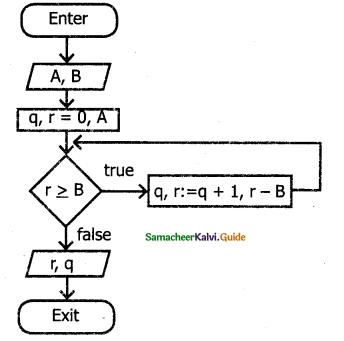Question 3.
Explain sequential statement with suitable example.
A sequential statement is composed of a sequence of statements. The statements in the sequence are executed one after another, in the same order as they are written in the algorithm, and the control flow is said to be sequential. Let S1 and S2 be statements. A sequential statement composed of S1 and S2 is written as
S1
S2
In order to execute the sequential statement, first, do S1 and then do S2.

The sequential statement given above can be represented in a flowchart as shown in in the following Figure. The arrow from S1 to S2 indicates that S1 is executed, and after that, S2 is executed.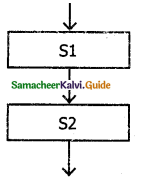Let the input property be P, and the input-output relation be Q, for a problem. If statement S solves the problem, it is written as
i) –P
ii) S
iii) — QQuestion 4.
Explain alternative statement with an example. A condition is a phrase that describes a test of the state. If C is a condition and both SI and S2 are statements, then

if C
S1
else
S2

is a statement, called an alternative statement, that describes the following action:
i) Test whether C is true Or false.
ii) If C is true, then do SI; otherwise do S2.

In pseudo-code, the two alternatives S1 and S2 are indicated by indenting them from the keywords if and else, respectively. Alternative control flow is depicted in the following flowchart.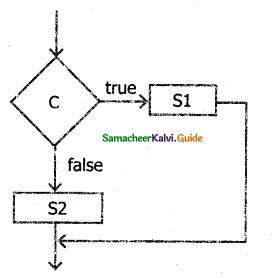Condition C has two outgoing arrows, labeled true and false. The true arrow points to the SI box. The false arrow points to the S2 box. Arrows out of S1 and S2 point to the same box, the box after the alternative statement.

Question 5.
Explain conditional statement with suitable example.
Sometimes we need to execute a statement only if a condition is true and do nothing if the condition is false. This is equivalent to the alternative statement in which the else-clause is empty. This variant of alternative statement is called a conditional statement. If C is a condition and S is a statement, then
if C
S
is a statement, called a conditional statement, that describes the following action:

• Test whether C is true or false.
• If C is true then do S; otherwise do nothing.

The conditional control flow is depicted in the following flowchart.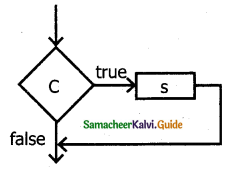Question 6.
Write a note on case analysis.
An alternative statement analyses the problem into two cases. The case analysis statement generalizes it to multiple cases. Case analysis splits the problem into an exhaustive set of disjoint cases. For each case, the problem is solved independently. If C1, C2, and C3 are conditions, and S1, S2, S3, and S4 are statements, a 4 – case analysis statement has the form.

1. case C1
2. S1
3. case C2
4. S2
5. case C3
6. S3
7. else
8. S4

The conditions C1, C2, and C3 are evaluated in turn. For the first condition that evaluates to true, the corresponding statement is executed, and the case analysis statement ends. If none of the conditions evaluates to true, then the default case S4 is executed.

1. The cases are exhaustive: at least one of the cases is true. If all conditions are false, the default case is true.
2. The cases are disjoint: only one of the cases is true. Though it is possible for more than one condition to be true, the case analysis always executes only one case, the first one that is true.
3. f the three conditions are disjoint, then the four cases are (1) C1, (2) C2, (3) C3, (4) (not C1), and (not C2) and (not C3).Question 7.
Explain iterative statement with suitable example.
An iterative process executes the same action repeatedly, subject to a condition C.
If C is a condition and S is a statement, then
while C
S
is a statement, called an iterative statement, that describes the following action:

• Test whether C is true or false.
• If C is true, then do S and go back to step 1; otherwise do nothing.

The iterative statement is commonly known as a loop. These two steps, testing C and executing S, are repeated until C becomes false. When C becomes false, the loop ends, and the control flows to the statement next to the iterative statement.

The condition C and the statement S are called the loop condition and the loop body, respectively. Testing the loop condition and executing the loop body once is called an iteration, not C is known as the termination condition.
Iterative control flow is depicted in the following flowchart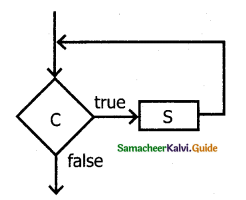Condition C has two outgoing arrows, true and false. The true arrow points to S box. If C is true, S box is executed and control flows back to C box. The false arrow points to the box after the iterative statement (dotted box). If C is false, the loop ends and the control flows to the next box after the loop.